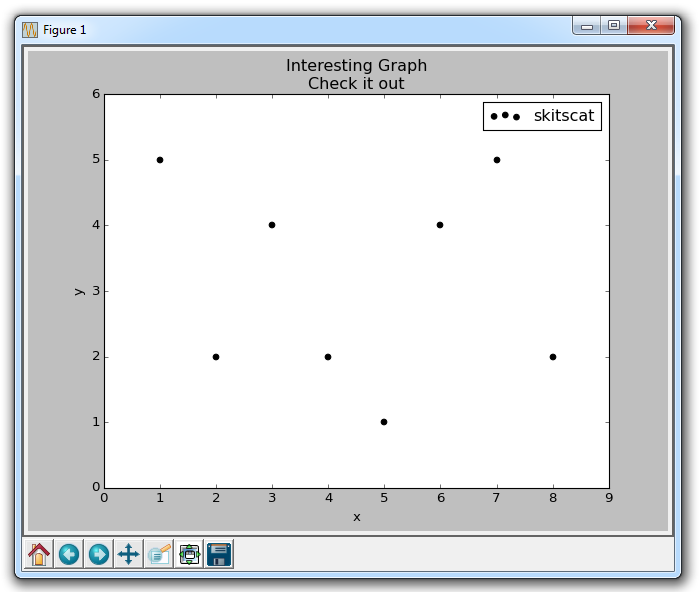## Scatter Plots with Matplotlib

Next up, we cover scatter plots! The idea of scatter plots is usually to compare two variables, or three if you are plotting in 3 dimensions, looking for correlation or groups.

Some sample code for a scatter plot:

```import matplotlib.pyplot as plt

x = [1,2,3,4,5,6,7,8]
y = [5,2,4,2,1,4,5,2]

plt.scatter(x,y, label='skitscat', color='k', s=25, marker="o")

plt.xlabel('x')
plt.ylabel('y')
plt.title('Interesting Graph\nCheck it out')
plt.legend()
plt.show()
```

The result:The plt.scatter allows us to not only plot on x and y, but it also lets us decide on the color, size, and type of marker we use. There are a bunch of marker options, see the Matplotlib Marker Documentation for all of your choices.

The next tutorial:• Introduction to Matplotlib and basic line

• Legends, Titles, and Labels with Matplotlib

• Bar Charts and Histograms with Matplotlib

• Scatter Plots with Matplotlib
• Stack Plots with Matplotlib

• Pie Charts with Matplotlib

• Data from the Internet for Matplotlib

• Converting date stamps for Matplotlib

• Basic customization with Matplotlib

• Unix Time with Matplotlib

• Colors and Fills with Matplotlib

• Spines and Horizontal Lines with Matplotlib

• Candlestick OHLC graphs with Matplotlib

• Styles with Matplotlib

• Live Graphs with Matplotlib

• Annotations and Text with Matplotlib

• Annotating Last Price Stock Chart with Matplotlib

• Subplots with Matplotlib

• Implementing Subplots to our Chart with Matplotlib

• More indicator data with Matplotlib

• Custom fills, pruning, and cleaning with Matplotlib

• Share X Axis, sharex, with Matplotlib

• Multi Y Axis with twinx Matplotlib

• Custom Legends with Matplotlib

• Basemap Geographic Plotting with Matplotlib

• Basemap Customization with Matplotlib

• Plotting Coordinates in Basemap with Matplotlib

• 3D graphs with Matplotlib

• 3D Scatter Plot with Matplotlib

• 3D Bar Chart with Matplotlib

• Conclusion with Matplotlib